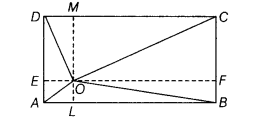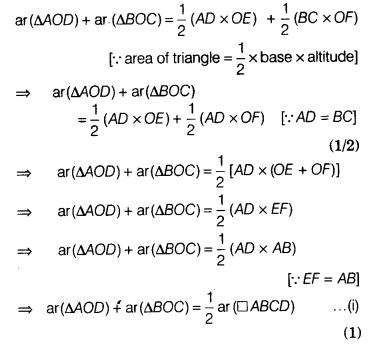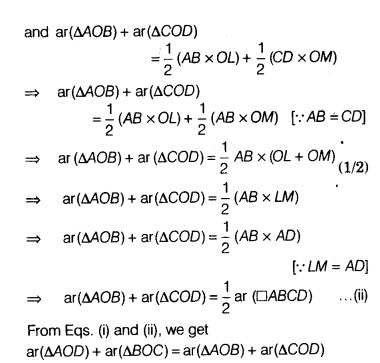# A point O inside a rectangle ABCD is joined to the vertices

.A point O inside a rectangle ABCD is joined to the vertices. Prove that the Sum of the areas of a pair of opposite triangles so formed is equal to the sum of the areas of other pair of triangles.

Given A rectangle ABCD and O is a point inside it. OA, OB, OC and OD have been joined.

To prove ar(∆AOD) + ar(∆bOC) = ar(∆AOB)+ar(∆COD)
Construction Draw EF|| AB and LM|| AD